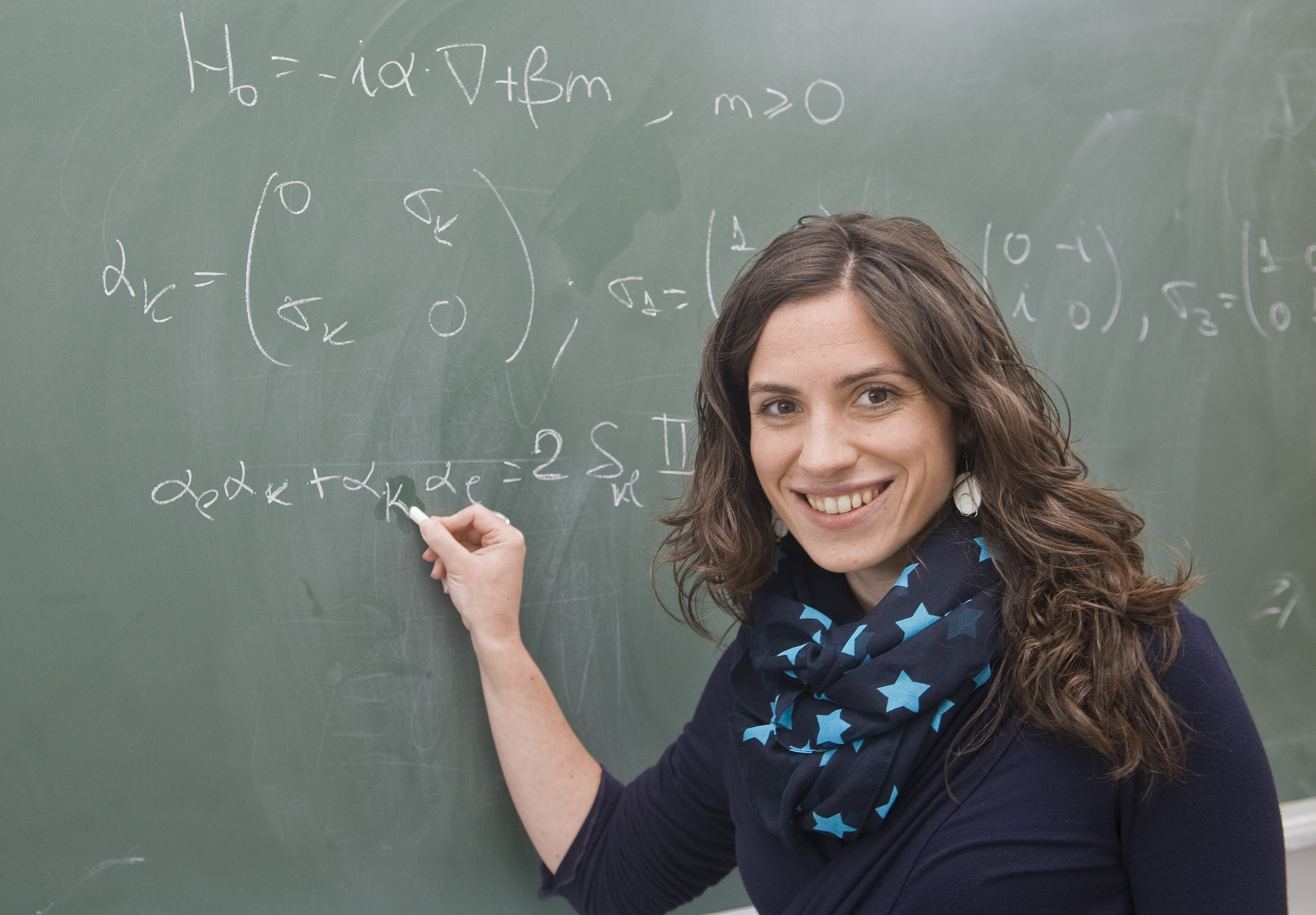# New attempt to solve an old equation

2013/01/18 Roa Zubia, Guillermo - Elhuyar Zientzia Iturria: Elhuyar aldizkariaNaiara Arrizabalaga, mathematician of the UPV Ed. UPV/EHU

The mathematician Naiara Arrizabalaga has sought solutions to the famous equation of Dirac, which describes the particles, in a doctoral thesis carried out in the UPV.

It is one of the most famous and complex physics equations proposed by the British physicist Paul Dirac to describe in 1928 the movement of particles with spin ½, like the electron. Since electrons move at high speed, it is very important to take into account the equations that describe them, since the effects of this theory are made at high speeds. Although earlier Schrödinger also found an equation describing the motion of the electron, it did not take into account the theory of relativity.

The equations of Dirac yes. But the complexity of the structure of the Dirac equation greatly hinders its analysis. According to mathematician Arrizabalaga, “less have been worked on the Dirac equation than on other partial derived equations such as waves or Schrödinger’s”.

Arrizabalaga's doctoral thesis analyzes Dirac's relativistic equation on the scarce work that has been done in this regard. The aim of the thesis is to solve what cannot be resolved. That is, to seek the solution to specific cases in which the solution is not mathematically accurate; specifically, Arrizabalaga studied the self-aggregated extensions of the operator Diracen applied to certain potentials, such as the electromagnetic potentials of singular origin, for which differences of the type Hardy-Dirac will be used. Complex mathematical resources to find solutions to an ancient but complex equation.eu es fr en ca gl

## Gai honi buruzko eduki gehiago

Elhuyarrek garatutako teknologia

1995-3-1Zientzia
1995-8-2Zientzia
##### Zer da antimateria?
2018-10-29Zientzia